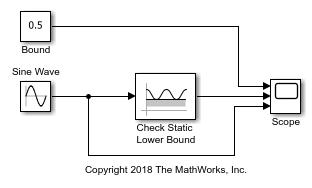# Check Signal Lower Bound with Check Static Lower Bound Block

Using the Check Static Lower Bound block, you can check if an input signal falls below a defined value.In this example, the Check Static Lower Bound block compares the value of a Sine Wave block to the value entered in the Lower bound parameter, which is `0.5`. The Constant block, labeled Bound, illustrates this parameter in the Scope block but does not affect the parameter value. The Check Static Lower Bound block checks if the value of the input signal is greater than the Lower bound. If it is, the block asserts `true (1)`. Because the Output assertion signal parameter of the block is selected, the block outputs the assertion value. Run the simulation to observe the model output.At the start of the simulation, the Check Static Lower Bound block outputs `0` because the value of the Sine Wave block is less than the bound. At a time of `0.52`, the sine wave exceeds `0.5`. The Check Static Lower Bound block recognizes this change and outputs `1`. When the time reaches `2.62`, the sine wave falls below the bound and the assertion is `false`. This pattern repeats until the simulation end time.